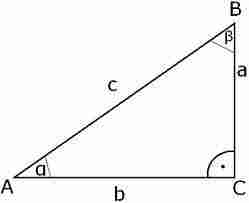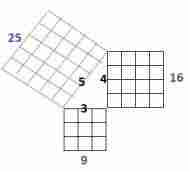# Pythagoras Theorem Calculation with examples

 Select side Hypotenuse side (c) Adjacent side (a) Opposite side (b) Adjacent side (a) = Opposite side (b) = Hypotenuse side (c) =
In a right angled triangle: the square of the hypotenuse is equal to the sum of the squares of the other two sides. Suppose right triangle with sides a and b and hypotenuse c then c2 = a2 + b2
Or c = √(a2 + b2)
Or a = √(c2 - b2)
Or b = √(c2 + a2)### Examples :

1. Find the hypotenuse where two sides a = 6 cm and b = 8 cm.

The formula for hypotenuse c = √(a2 + b2)
= √(62 + 82)
= √(6X6 + 8x8)
= √(36 + 64)
= √(100)
=10 cm

2. Find the hypotenuse where two sides a = 4 and b = 3.

The formula for hypotenuse c = √(a2 + b2)
= √(42 + 32)
= √(4X4 + 3x3)
= √(16 + 9)
= √(25)
=5